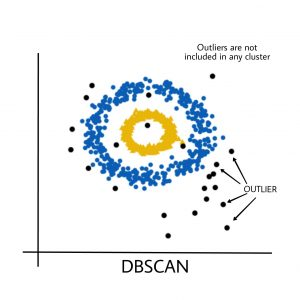2 views

I have a scenario where I have several thousand instances of data. The data itself is represented as a single integer value. I want to be able to detect when an instance is an extreme outlier.

For example, with the following example data:

a = 10

b = 14

c = 25

d = 467

e = 12

d is clearly an anomaly, and I would want to perform a specific action based on this.

I was tempted to just try to use my knowledge of the particular domain to detect anomalies. For instance, figure out the distance from the mean value that is useful, and check for that, based on heuristics. However, I think it's probably better if I investigate more general, robust anomaly detection techniques, which have some theory behind them.

Since my working knowledge of mathematics is limited, I'm hoping to find a technique that is simple, such as using standard deviation. Hopefully, the single-dimensioned nature of the data will make this quite a common problem, but if more information for the scenario is required please leave a comment and I will give more info.

by (33.1k points)
edited by

There are many ways to solve your problem. Here you can check some common techniques to deal with this problem:

Three-sigma rule:

mu  = mean of the data

std = standard deviation of the data

IF abs(x-mu) > 3*std  THEN x is outlier

IQR outlier test:

Q25 = 25th_percentile

Q75 = 75th_percentile

IQR = Q75 - Q25         // interquartile range

IF (x < Q25 - 1.5*IQR) OR (Q75 + 1.5*IQR < x) THEN  x is a mild outlier

IF (x < Q25 - 3.0*IQR) OR (Q75 + 3.0*IQR < x) THEN  x is an extreme outlierDBSCAN:

A better-suited technique is the DBSCAN: a density-based clustering algorithm. Basically, it grows regions with sufficiently high density into clusters which will be a maximal set of density-connected points.For example:

>>> from sklearn.cluster import DBSCAN

>>> import numpy as np

>>> X = np.array([[1, 2], [2, 2], [2, 3],

...               [8, 7], [8, 8], [25, 80]])

>>> clustering = DBSCAN(eps=3, min_samples=2).fit(X)

>>> clustering.labels_

array([ 0,  0, 0, 1, 1, -1])

I hope it helps.